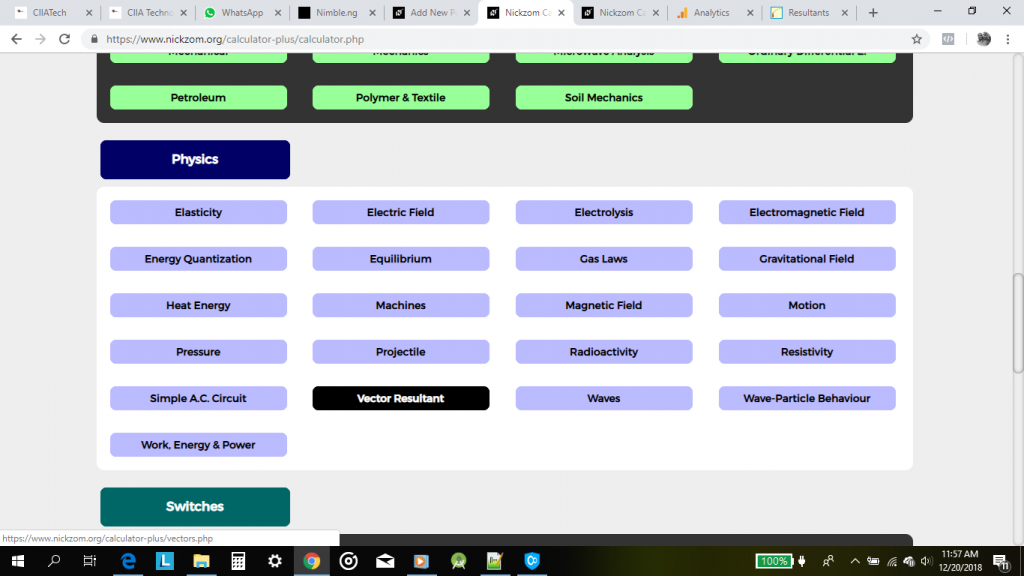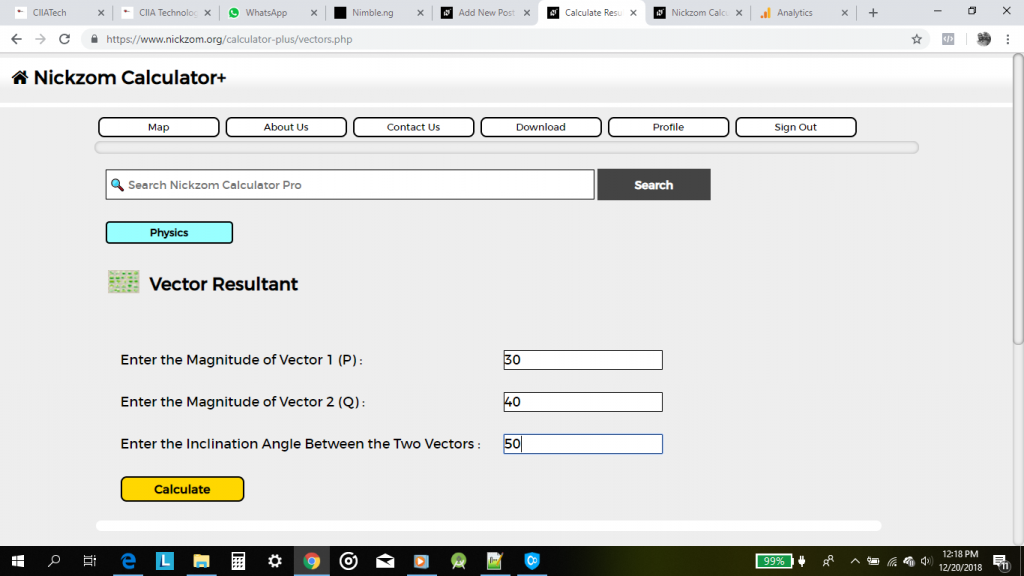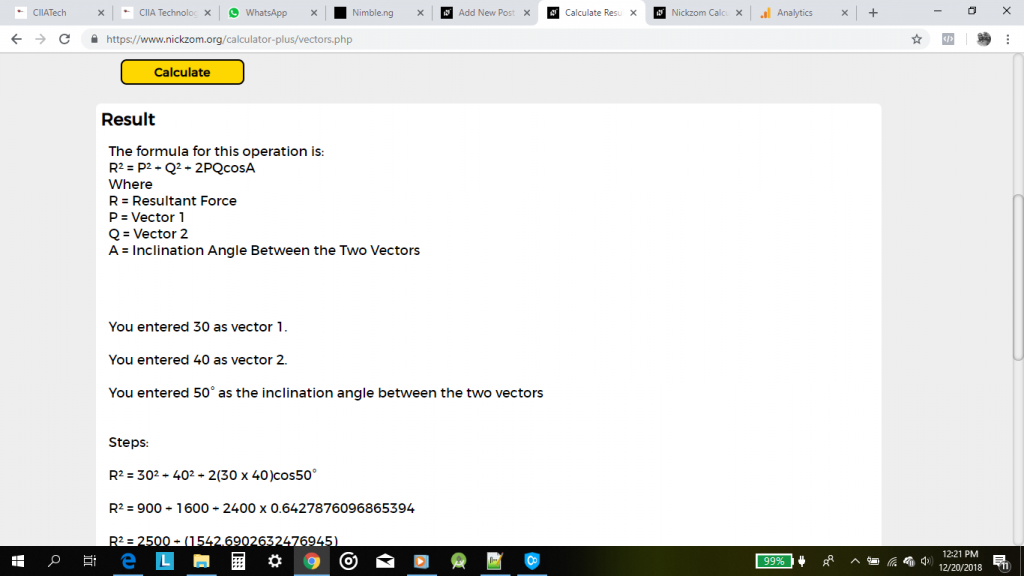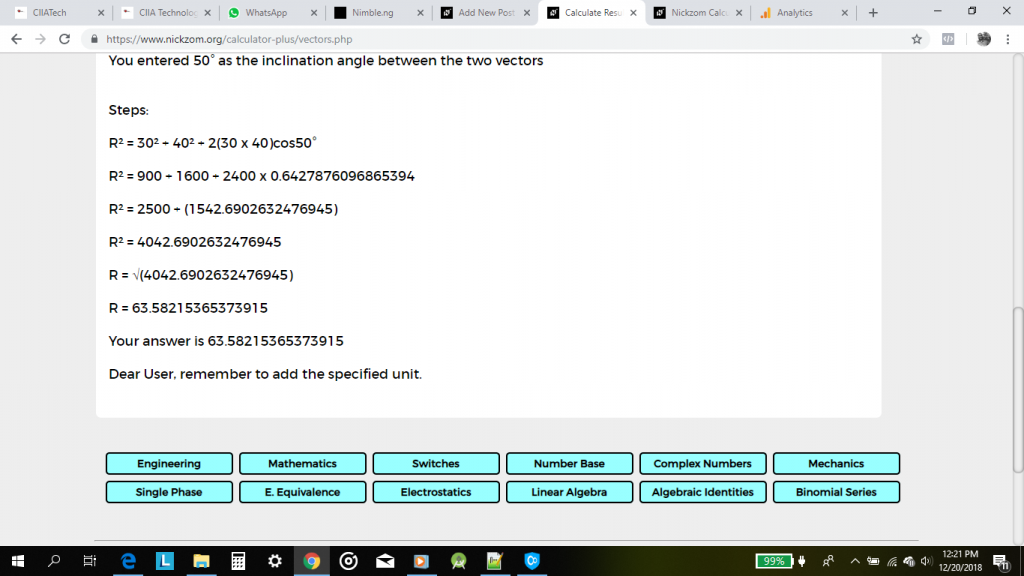# How to Calculate the Resultant of Two Vectors (Physics)

According to Math Warehouse,

The resultant vector is the vector that ‘results’ from adding two or more vectors together.

The formula for calculating the resultant of two vectors is:

R = √[P2 + Q2 + 2PQcosθ]

Where:
R = Resultant of the Two Vectors
P = Magnitude of the First Vector
Q = Magnitude of the Second Vector
θ = Inclination Angle between the Two Vectors

Let’s solve an example, find the resultant of two vectors where the first vector has a magnitude of 30 and the second vector has a magnitude of 40 with 50° has the inclination between the two vectors.

From the example above, we can see that P is 30, Q is 40 and θ is 50. Now, inserting these values into the formula we compute for the resultant of the two vectors.

R = √[(30)² + (40)² + 2(30)(40) . cos50°]
R = √[900 + 1600 + 2(1200) . (0.6427876096865394)]
R = √[900 + 1600 + 2400(0.6427876096865394)]
R = √[900 + 1600 + 1542.6902632476945]
R = √[4042.6902632476945]
R = 63.58215365373915

Therefore, the resultant of the two vectors is 63.58215365373915.

To be able to get the resultant of two vectors using Nickzom Calculator. You need to know the magnitude of the two vectors and the inclination between the two vectors.

Nickzom Calculator is the very first calculator encyclopedia to come out from Nigeria. It is capable of solving over 50,000 calculations and presenting the steps (workings). Fortunately, Nickzom Calculator can compute conception and best intercourse dates.

You can access Nickzom Calculator via any of these means:
Web – https://www.nickzom.org/calculator-plus
Apple (Paid) – https://itunes.apple.com/us/app/nickzom-calculator/id1331162702?mt=8

Once, you have access to Nickzom Calculator proceed to the Calculator Map, click on Vector Resultant under Physics sectionClicking on the Vector Resultant, displays the page for you to enter the values for each of the parameters.Now, enter the values of the parameters: P = 30, Q = 40 and θ = 50Now, click on CalculateNickzom Calculator solves for the resultant of the two vectors and shows you the formula, workings and answer.

Peace!